Home | | Business Maths 12th Std | Exercise 5.2: Interpolation

# Exercise 5.2: Interpolation

Book back answers and solution for Exercise questions - Maths: Numerical Methods: Interpolation: Problem Questions with Answer, Solution

Exercise 5.2

1. Using graphic method, find the value of y when x = 48 from the following data: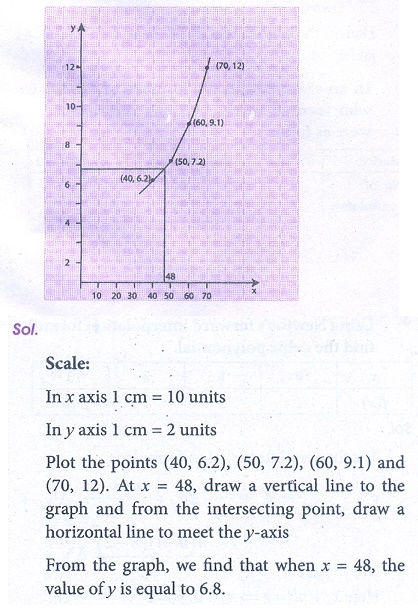2. The following data relates to indirect labour expenses and the level of outputEstimate the expenses at a level of output of 350 units, by using graphic method.3. Using NewtonŌĆÖs forward interpolation formula find the cubic polynomial.4. The population of a city in a censes taken once in 10 years is given below. Estimate the population in the year 1955.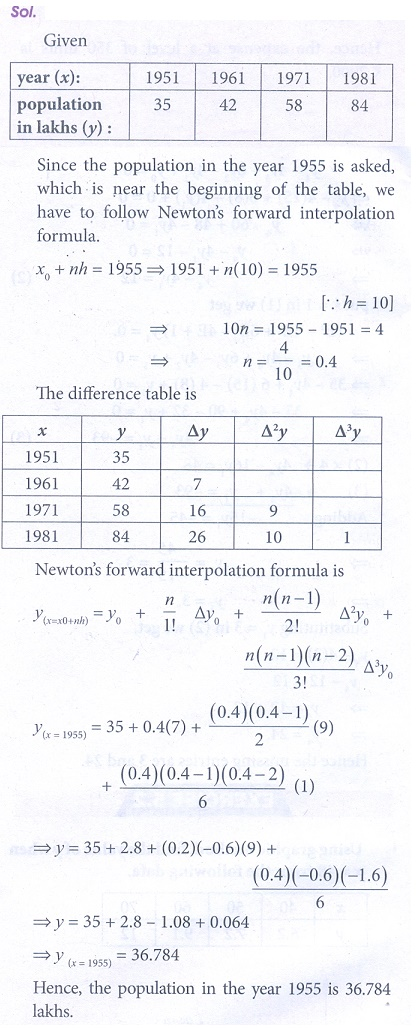5. In an examination the number of candidates who secured marks between certain interval were as follows:Estimate the number of candidates whose marks are lessthan 70.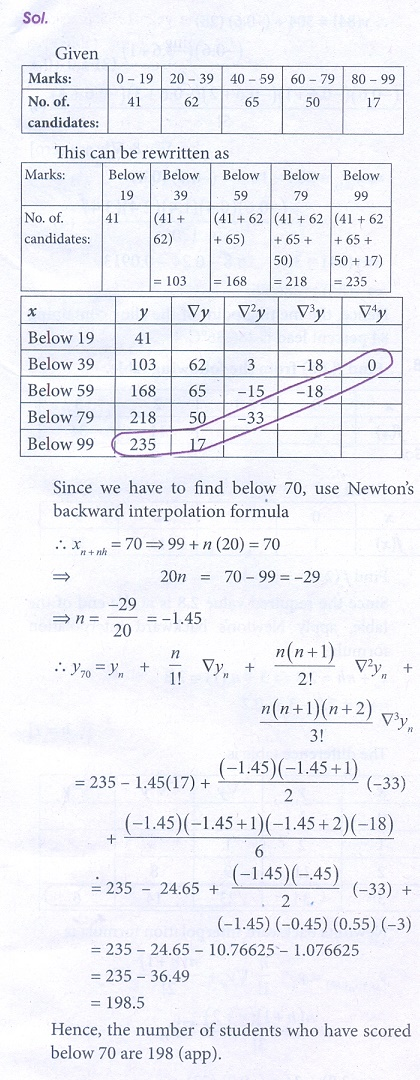6. Find the value of f ( x) when x = 32 from the following table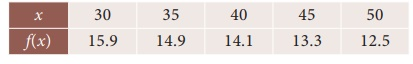7. The following data gives the melting point of a alloy of lead and zinc where ŌĆśtŌĆÖ is the temperature in degree c and P is the percentage of lead in the alloyFind the melting point of the alloy containing 84 percent lead.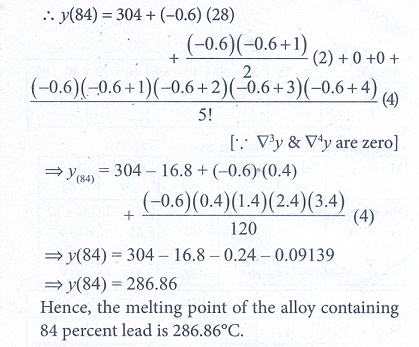8. Find f(2.8) from the following table.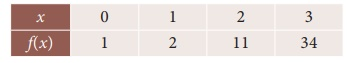9. Using interpolation estimate the output of a factory in 1986 from the following data10. Use LagrangeŌĆÖs formula and estimate from the following data the number of workers getting income not exceeding Rs. 26 per month.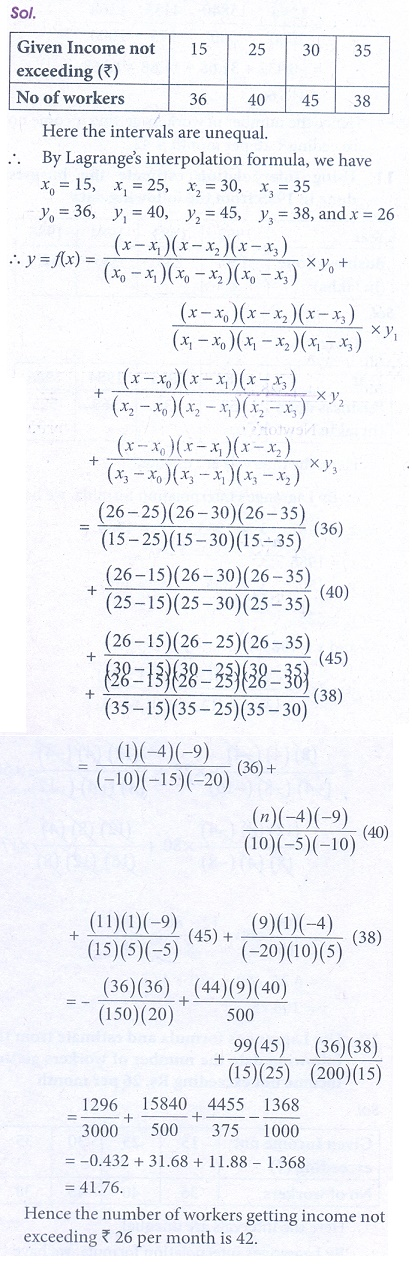11. Using interpolation estimate the business done in 1985 from the following data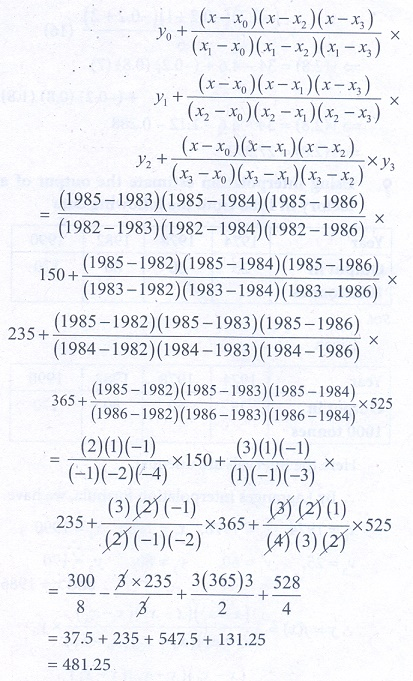12. Using interpolation, find the value of f(x) when x = 15